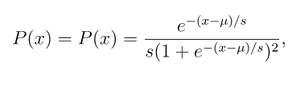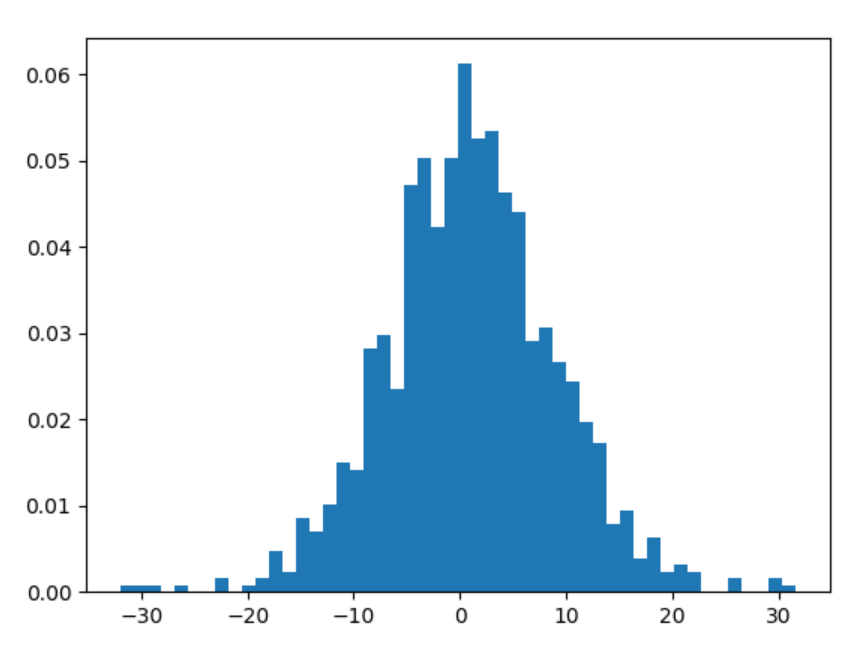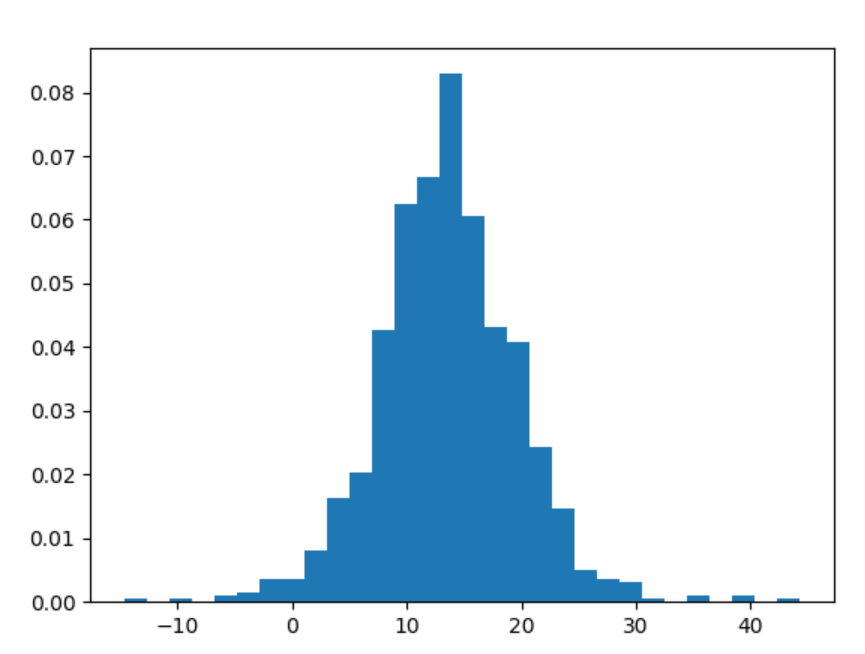# numpy.random.logistic() in Python

• Last Updated : 15 Jul, 2020

With the help of numpy.random.logistic() method, we can get the random samples of logistic distribution and returns the random samples by using this method.logistic distribution

Syntax : numpy.random.logistic(loc=0.0, scale=1.0, size=None)

Return : Return the random samples as numpy array.

Example #1 :

In this example we can see that by using numpy.random.logistic() method, we are able to get the random samples of logistic distribution and return the random samples by using this method.

## Python3

 `# import numpy``import` `numpy as np``import` `matplotlib.pyplot as plt`` ` `# Using numpy.random.logistic() method``gfg1 ``=` `np.random.logistic(``1.23``, ``3.14``, ``1000``)``gfg2 ``=` `np.random.logistic(gfg1, ``3.14``, ``1000``)`` ` `count, bins, ignored ``=` `plt.hist(gfg2, ``50``, density ``=` `True``)``plt.show()`

Output :Example #2 :

## Python3

 `# import numpy``import` `numpy as np``import` `matplotlib.pyplot as plt`` ` `# Using numpy.random.logistic() method``gfg ``=` `np.random.logistic(``13.31``, ``3.31``, ``1000``)`` ` `count, bins, ignored ``=` `plt.hist(gfg, ``30``, density ``=` `True``)``plt.show()`

Output :My Personal Notes arrow_drop_up